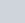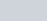If two opposite vertices of a square are (5, 4)
Question:

If two opposite vertices of a square are (5, 4) and (1, −6), find the coordinates of its remaining two vertices.

Solution:

The distance $d$ between two points $\left(x_{1}, y_{1}\right)$ and $\left(x_{2}, y_{2}\right)$ is given by the formula

$d=\sqrt{\left(x_{1}-x_{2}\right)^{2}+\left(y_{1}-y_{2}\right)^{2}}$

In a square all the sides are of equal length. The diagonals are also equal to each other. Also in a square the diagonal is equal totimes the side of the square.

Here let the two points which are said to be the opposite vertices of a diagonal of a square be A(5,4) and C(1,6).

Let us find the distance between them which is the length of the diagonal of the square.

$A C=\sqrt{(5-1)^{2}+(4+6)^{2}}$

$=\sqrt{(4)^{2}+(10)^{2}}$

$=\sqrt{16+100}$

$A C=2 \sqrt{29}$

Now we know that in a square,

Side of the square $=\frac{\text { Diagonal of the square }}{\sqrt{2}}$

Substituting the value of the diagonal we found out earlier in this equation we have,

Side of the square $=\frac{2 \sqrt{29}}{\sqrt{2}}$

Side of the square $=\sqrt{58}$

Now, a vertex of a square has to be at equal distances from each of its adjacent vertices.

Let P(x, y) represent another vertex of the same square adjacent to both ‘A’ and ‘C’.

$A P=\sqrt{(5-x)^{2}+(4-y)^{2}}$

$C P=\sqrt{(1-x)^{2}+(-6-y)^{2}}$

But these two are nothing but the sides of the square and need to be equal to each other.

$A P=C P$

$\sqrt{(5-x)^{2}+(4-y)^{2}}=\sqrt{(1-x)^{2}+(-6-y)^{2}}$

Squaring on both sides we have,

$(5-x)^{2}+(4-y)^{2}=(1-x)^{2}+(-6-y)^{2}$

$25+x^{2}-10 x+16+y^{2}-8 y=1+x^{2}-2 x+36+y^{2}+12 y$

$8 x+20 y=4$

$2 x+5 y=1$

From this we have, $x=\frac{1-5 y}{2}$

Substituting this value of ‘x’ and the length of the side in the equation for ‘AP’ we have,

$A P=\sqrt{(5-x)^{2}+(4-y)^{2}}$

$\sqrt{58}=\sqrt{(5-x)^{2}+(4-y)^{2}}$

Squaring on both sides,

$58=(5-x)^{2}+(4-y)^{2}$

$58=\left(5-\left(\frac{1-5 y}{2}\right)\right)^{2}+(4-y)^{2}$

$58=\left(\frac{9+5 y}{2}\right)^{2}+(4-y)^{2}$

$58=\frac{81+25 y^{2}+90 y}{4}+16+y^{2}-8 y$

$232=81+25 y^{2}+90 y+64+4 y^{2}-32 y$

$87=29 y^{2}+58 y$

We have a quadratic equation. Solving for the roots of the equation we have,

$29 y^{2}+58 y-87=0$

$29 y^{2}+87 y-29 y-87=0$

$29 y(y+3)-29(y+3)=0$

$(y+3)(29 y-29)=0$

$(y+3)(y-1)=0$

The roots of this equation are −3 and 1.

Now we can find the respective values of ‘x’ by substituting the two values of ‘y

When$x=\frac{1-5(-3)}{2}$

$=\frac{1+15}{2}$

$x=8$

When $y=1$

$x=\frac{1-5(1)}{2}$

$=\frac{1-5}{2}$

$x=-2$

Therefore the other two vertices of the square are $(8,-3)$ and $(-2,1)$.